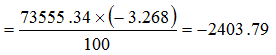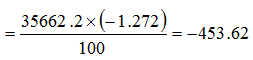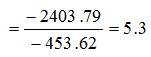Answer question nos. 1 – 3 based on the following information:
Given below are a few data points on the Indian economy from 2005 to 2010:Per capital GDP is arrived by dividing GDP by population.

Question 1.  What is the ratio of the current account balance in 2010 to the current account balance in 2005?
A. 0.35
B. 4.56
C. 5.01
D. 2.57
E. 5.30

Question 2. Read the statements given below:
1. Exports were more than imports in 2006
2. Imports were more than exports in 2009
3. Exports increased at faster rate than imports during the period 2005 to 2010
Which of the above statements is necessarily true?
A. 1 and 2
B. 1,2 and 3
C. 3 only
D. 2 only
E. 1 only

Question 3. Read the statements given below:
1. Exports were more than imports in 2006
2. Imports were more than exports in 2009
3. Exports increased at faster rate than imports during the period 2005 to 2010
Which of the above statements is necessarily true?
A. 1 and 2
B. 1,2 and 3
C. 3 only
D. 2 only
E. 1 only

Current account balance in 2010Current account balance in 2005∴   Required ratioNothing can be said about statements 1 and 2 as the absolute values of exports and imports cannot be determined. Imports decreased during the period 2005 to 2010. Hence, only statement 3 is true.